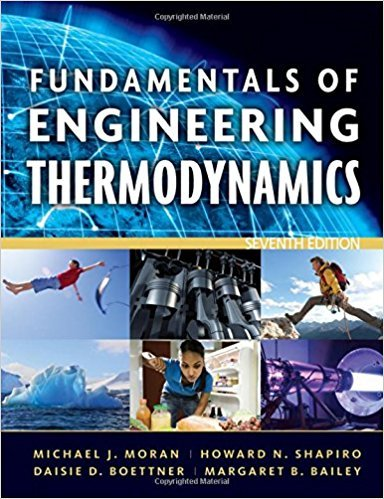×
Get Full Access to Fundamentals Of Engineering Thermodynamics - 7 Edition - Chapter 2 - Problem 56p
Get Full Access to Fundamentals Of Engineering Thermodynamics - 7 Edition - Chapter 2 - Problem 56p

×

# Using the Energy BalanceAs shown in Fig. P2.56, a gasISBN: 9780470495902 50

## Solution for problem 56P Chapter 2

Fundamentals of Engineering Thermodynamics | 7th Edition

• Textbook Solutions
• 2901 Step-by-step solutions solved by professors and subject experts
• Get 24/7 help from StudySoup virtual teaching assistantsFundamentals of Engineering Thermodynamics | 7th Edition

4 5 1 419 Reviews
30
3
Problem 56P

Problem 56P

Using the Energy Balance

As shown in Fig. P2.56, a gas contained within a piston-cylinder assembly, initially at a volume of 0.1 m3, undergoes a constant-pressure expansion at 2 bar to a final volume of 0.12 m3, while being slowly heated through the base. The change in internal energy of the gas is 0.25 kJ. The piston and cylinder walls are fabricated from heat-resistant material, and the piston moves smoothly in the cylinder. The local atmospheric pressure is 1 bar.

(a) For the gas as the system, evaluate work and heat transfer, each in kJ.

(b) For the piston as the system, evaluate work and change in potential energy, each in kJ.Fig. P2.56

Step-by-Step Solution:

(a)

Step 1 of 3

We have to evaluate work and heat transfer for the gas as the system.

The work done for the gas as the system can be evaluated using the expressionwhere,constant pressure = 2 bar =N/m2= change in volume in m3

The change in volume of the gas isThus, the work done for the gas as the system isStep 2 of 3

Step 3 of 3

##### ISBN: 9780470495902

Unlock Textbook Solution